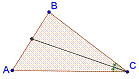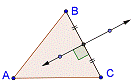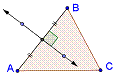## Wednesday, February 29, 2012

### Tangent Circles

This is a Java Applet created using GeoGebra from www.geogebra.org - it looks like you don't have Java installed, please go to www.java.com

## Monday, February 27, 2012

### Triangle Centers - II

Exploring Triangle Centres Using Geogebra
Visualizing Triangle Centers Using Geogebra
(Part-2)

In my previous post http://mathematicsbhilai.blogspot.in/2012/02/triangle-centers-i.html , I dicussed about Centroid and Circumcenter of a triangle. In this post we will discuss about Incenter and Orthocenter of a triangle. Further we will discuss about Euler Line and Nine Point Circle.
a.      Incenter
An angle bisector of a triangle is a line segment that bisects an angle of the triangle.Figure – 1     Angle Bisectors

There are three angle bisectors of a triangle.

Three angle bisectors of a triangle meet at a point or they are concurrent. This point is called incenter of the triangle. It is called the incenter because it is the centre of the circle inscribed (the largest circle that will fit inside the triangle)  in the triangle.

Centroid of triangle always remains inside the triangle irrespective of its type (scalene , isosceles or equilateral)

Figure – 2    Incenter  (I) of a  triangle

b.      Orthocenter
The altitude of a triangle is a line which passes through a vertex of the triangle and is perpendicular to the opposite side. There are therefore three altitudes possible, one from each vertex.

Figure – 3    Altitudes of a Triangle

AF , BE and CF are three altitudes of  triangle ABC.
The altitudes (perpendiculars from the vertices to the opposite sides) of a triangle meet at a point  i.e. they are concurrent . This point is called orthocenter of the triangle.

For an acute triangle the orthocenter is inside the triangle, for obtuse triangle it lies outside and for a right triangle it lies at the vertex of the triangle where right angle is formed.

Figure – 4  Orthocenter (O) of an acute triangle

There is a very interesting fact  , If the orthocenter of triangle ABC is O, then the orthocenter of triangle OBC is A, the orthocenter of  triangle OCA is B and the orthocenter of triangle OAB is C.

Figure – 5   Orthocenter (O) of an obtuse triangle

Figure – 6   Orthocenter (O) of a right triangle

c.       Euler Line
The orthocenter O, the circumcenter C, and the centroid G of any triangle are collinear. Furthermore, G is between O and C (unless the triangle is equilateral, in which case the three points coincide) and OG = 2GC.   The line through O, C, and G is called the Euler line of the triangle.

Figure – 7   Euler Line

d.      Nine Point Circle

If DABC is any triangle, then the midpoints of the sides of DABC, the feet of the altitudes of DABC, and the midpoints of the segments joining the orthocenter of DABC to the three vertices of DABC all lie on  single circle and this circle is called the nine point circle.

Centre of nine point circle always lies on the Euler line , and is the mid point of the line segment joining orthocentre and circumcentre.

In an equilateral triangle ,  the Orthocenter, centroid, and circumcenter  conicide, so that the Euler line has a length of 0. Further, the altitiudes and medians are concurrent, so the 9-point circle now contains only 6 points.

Figure – 8  Nine Point Circle  (Scalene Triangle)

Nine Point Circle in an Isosceles Triangle
In an isosceles triangle the Euler line is collinear with the median from the vertex to  the base. The altitude and perpendicular bisectors to the base are  the same, so the intersection of those two lines with the base of the triangle is a coincident point.  Thus our 9-point circle intersects 8 distinct points.  The obtuse isosceles triangle also has  8 points in its 9-point circle.

Figure – 9  Nine Point Circle  (Isosceles Triangle)

## Saturday, February 25, 2012

### Triangle Tessellation

This is a Java Applet created using GeoGebra from www.geogebra.org - it looks like you don't have Java installed, please go to www.java.com

## Thursday, February 23, 2012

### Snowflakes

This is a Java Applet created using GeoGebra from www.geogebra.org - it looks like you don't have Java installed, please go to www.java.com

## Monday, February 20, 2012

### Algebraic Identity - Difference of Squares

This is a Java Applet created using GeoGebra from www.geogebra.org - it looks like you don't have Java installed, please go to www.java.com

## Saturday, February 18, 2012

### Algebraic Identity - Square of Differences

This is a Java Applet created using GeoGebra from www.geogebra.org - it looks like you don't have Java installed, please go to www.java.com

## Friday, February 17, 2012

### Algebraic Identity - Square of Sum

This is a Java Applet created using GeoGebra from www.geogebra.org - it looks like you don't have Java installed, please go to www.java.com

## Thursday, February 16, 2012

### Doubling a Square

This is a Java Applet created using GeoGebra from www.geogebra.org - it looks like you don't have Java installed, please go to www.java.com

## Tuesday, February 14, 2012

### An Interesting Problem

This is a Java Applet created using GeoGebra from www.geogebra.org - it looks like you don't have Java installed, please go to www.java.com

## Monday, February 13, 2012

### Triangle Centers - I

Visualizing Triangle Centers Using Geogebra

(Part-1)
1. Introduction
Mark  three  non-collinear  point  P,  Q  and  R  on  a  paper.  Join  these  pints  in  all possible ways. The segments are PQ, QR and RP. A simple close curve formed by these three segments is called a triangle.

A triangle is a 3-sided polygon. Every triangle has three sides and three angles, some of which may be have equal measurements. The sides of a triangle are given special names in the case of a right triangle, the side opposite to the right angle is called the hypotenuse and the other two sides being known as the legs. All triangles are convex. The portion of the plane enclosed by the triangle is called the triangle interior, while the remainder is the exterior.

Some basic facts about a triangle are
• Construction of a triangle is possible only when the sum of lengths of two sides is greater than the third side.
• The sum of angles in a triangle is 180°.
• In a triangle if any of the two sides are equal then  angles opposite to equal sides are also equal.  Such triangles are called isosceles triangles.
• In a triangle  if three sides are equal , then the three interior angles are equal. Such triangles are called equilateral triangles.
• If a triangle has its three sides of unequal lengths then it is called a scalene triangle.
1. Introduction to Geogebra
GeoGebra is an educational software for exploring and demonstrating Geometry and Algebra. It is an open source application and is freely available.  It is capable of representing mathematical objects (at present 2-dimensional) algebraically and geometrically. For example, f(x) = x2 – 4x+2, a quadratic function, is represented by a parabola in graphical view and by an equation in algebraic window. A variable in Geogebra is represented by a slider. You can draw an object with the help of tools or by entering command in the input bar of GeoGebra window.
1. Centers of Triangle
On every triangle there are points where special lines or circles intersect, and those points usually have very interesting geometrical properties. Such points are called triangle centers. Some examples of triangle centers are incenter, orthocenter, centroid, circumcenter, excenters, Feuerbach point, Fermat points, etc.

Concurrent Lines :  Three lines are concurrent if there is a point P such that P lies on all three of the lines. The point P is called the point of concurrency. Three segments are concurrent if they have an interior point in common.

Two arbitrary lines will intersect in a point—unless the lines happen to be parallel. It is rare that three lines should have a point in common. One of the surprising and beautiful aspects of advanced Euclidean geometry is the fact that so many triples of lines determined by triangles are concurrent. Each of the triangle centers in this chapter is an example of that phenomenon.
1. Centroid
A median of a triangle is a line segment that joins any vertex of the triangle to the mid point of the opposite side. There are three medians of a triangle. Three medians of the triangle meet at a point i.e. they are concurrent . This point of concurrency is knows as the centroid of the triangle.

Each median divides the triangle into two smaller triangles of equal areas.

One of the basic ideas known about the centroid is that it it divides the medians into a 2:1 ratio.  The part of the median near to the vertex is always twice as long as the part near the midpoint of the side. If the coordinates of the triangle are known, then the coordinates of the centroid are the averages of the coordinates of the vertices.  If we call the three vertices A(x1,y1) , B(x2,y2) and C(x3,y3) then the coordinates of the centroid are

In the following figure – 1 , points D , E and F are respectively the mid points of sides BC , CA and AB of triangle ABC. AD , BE and CF are the medians of triangle and G is its centroid.

Centroid of triangle always remains inside the triangle irrespective of its type (scalene , isosceles or equilateral)Figure -1 Centroid (G) of a Triangle
1. Circumcenter
A  perpendicular bisector of a side of a triangle is a line which is perpendicular to the side and also passes through its mid point. There are three perpendicular bisectors of a triangle.Figure – 2    Perpendicular Bisectors of a Triangle

Three perpendicular bisectors of a triangle meet at a point i.e. they are concurrent. This point is called circumcenter of the triangle. It is called circumcenter because it is the centre of the circle circumscribing the triangle. (a circle passing through the three vertices of the triangle). The distance of circumcenter from three vertices is equal and is the radius of the circumcircle.

For an acute triangle the circumcenter is inside the triangle , for obtuse triangle it lies outside and for a right triangle it lies at the mid point of the hypotenuse of the triangle.

Figure – 3 Circumcenter (C ) of an acute triangle

Figure – 4 Circumcenter (C ) of an obtuse triangle

Figure – 5 Circumcenter (C ) of a right  triangle